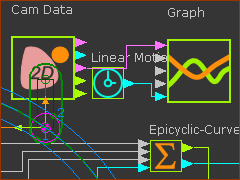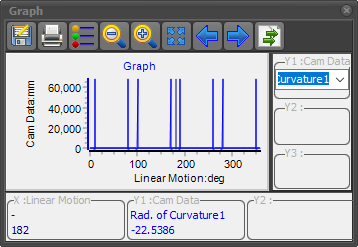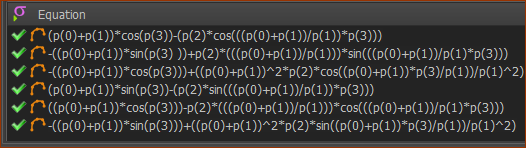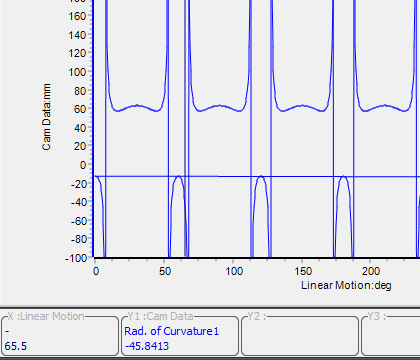﻿ Getting Started Tutorials - MechDesigner > Tutorial 18: Math FB > Step 18.3: Acceleration: Find the Radius-of-Curvature of the Cam

# Step 18.3: Acceleration: Find the Radius-of-Curvature of the Cam

### Plot the Radius-of-Curvature for the Cam we have found for the Epitrochoid Curve

A plot of a curve's Radius-of-Curvature is a good example because Radius-of-Curvature needs the Velocity and Acceleration Equations for the X and Y coordinates.

We must symbolically differentiate the Velocity Equations, given in Step 18.2, to find the Acceleration Equations.

Remember, MechDesigner does these calculations automatically with Gear-Pair. We are doing this with a Math FB only as an example of how to use the Math FB.Before we can plot the Radius-of-Curvature for a 2D-Cam, we must add a Cam-Data FB

 1.Mechanism-Editor : Click Machine elements toolbar > Add Cam-Data FB , then click the graphic-area 2.Mechanism-Editor : Open the Cam-Data dialog-box , then click the 2D-Cam. Close the Cam-Data dialog-box The 2D-Cam is now linked with the Cam-Data FB 3.Mechanism-Editor : Click Kinematic FB toolbar > Add a Graph FB, then click the graphic-area. 4.Mechanism-Editor : Drag a wire from the Radius-of-Curvature to the Graph input-connector.We can see graph for the Radius-of-Curvature is nonsense.

#### The Parametric-Equations for the X-axis and Y-axis Acceleration Components

 Differentiate the X and Y Parametric Velocity Equations for the Epitrochoid-Curve with respect to Θ. These are the two Parametric Equations for the X and Y Velocity Components: PXacc = -(a+b) * cos(Θ) + ( (a+b)2 * h * cos((a+b) * Θ / b) ) / b2 PYacc = -(a+b) * sin(Θ) + ( (a+b)2 * h * sin((a+b) * Θ / b) ) / b2 As before we must replace: a with p(0) ; b with p(1) ; h with p(2) ; Θ with p(3) In the Math FB they are: –((p(0)+p(1))*cos(p(3)))+((p(0)+p(1))^2 * p(2) * cos((p(0)+p(1)) * p(3) / p(1)) / p(1)^2) -((p(0)+p(1)) * sin(p(3))) + (( p(0)+p(1))^2 * p(2) * sin((p(0)+p(1)) * p(3) / p(1)) / p(1)^2)

#### Enter the Parametric Equations for the Acceleration Components in the Math FBThese are the 6 equations for the two output-connectors As entered the Math FB: •Equations 1, 2, 3: Position and Velocity, and Acceleration Equations for the X -axis •Equations 4, 5, 6: Position, Velocity, and Acceleration Equations for the Y-axis

#### Look again at the Plot for Radius-of-CurvatureWe can now see the the plot for the Radius-of-Curvature is as we expect. How can we check that the result is correct? We can check the results if we add a Circles to the Base-Part with a radius equal to the values given in the graph.